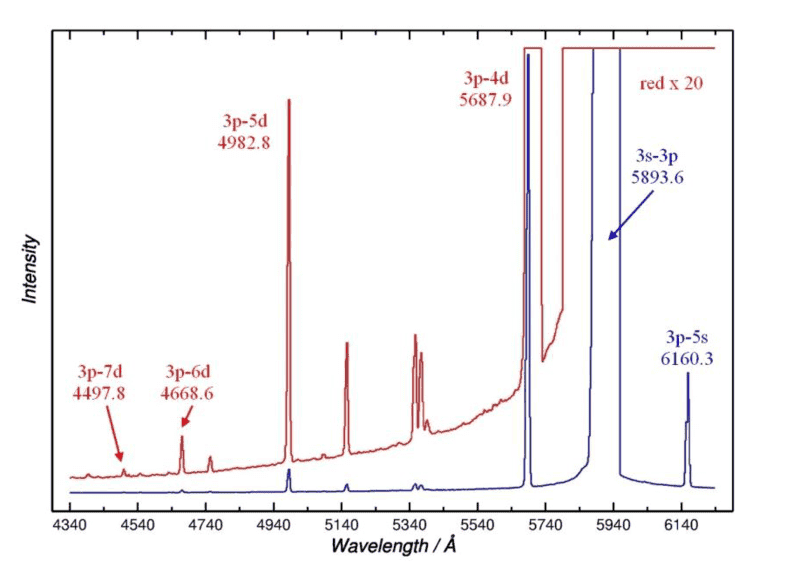# Quantum defect

## Homework Statement

The spectrum shows the series 3p - nd, n = 4 - 7 in Na as well as the resonance line 3s - 3p, with the experimental vacuum wavelengths in Å.

Calculate the quantum defect for the nd ##^2D## n = 4-7 terms. Estimate, as accurately as possible, the wavelength for 3p - 8d. The ionization energy in Na I is 41449.6 cm-1. Neglect all finestructure.

## Homework Equations

##E_{ionization} - E_{excitation} = T = R\frac{(z-N_{inner})^2}{(n-\delta)^2}##

## The Attempt at a Solution

Hi!

I can take the inverse of the given wavelength, to get T. ##\frac{1}{\lambda} = T##.

Then I can plug this into the above equation and solve for delta. ##\delta = n - sqrt(\frac{R*(z-N_{inner})^2)}{T})##

But my question is. Do I also need to take the quantum defect for p into account?

Where my ##T = R*(z-N_{inner})^2 ( \frac{1}{(n-\delta_p)^2} - \frac{1}{(n-\delta_d)^2}) ##

And if so, how would I get ##\delta_p##?

If it was s, I could impy solve for ##delta_s## when putting ##T= E_{io}##.

Figure attached#### Attachments

DrClaude
Mentor
But my question is. Do I also need to take the quantum defect for p into account?
From the data you have, can't you calculate E(3p) and then find the quantum defect from energy levels relative to that?

•Philip Land
From the data you have, can't you calculate E(3p) and then find the quantum defect from energy levels relative to that?

Thanks. I actually think I can by looking at the blue lines. I can put 6140 = ##R( \frac{1}{(5- \delta_s)^2}-\frac{1}{(3-\delta_p)^2})## Where quantum defect for s is ##\delta_s = 3- sqrt( \frac{R}{E_{io}}.## I guess.

On the right track? :)

There's no facit so I can't really tell if the asnswer is correct.

From the data you have, can't you calculate E(3p) and then find the quantum defect from energy levels relative to that?
But doing this i get

delta p = 2.739

delta s = 1.373

delta d = 3.1356

Which seems to be to high values.